Industry White Papers

# Switching Inductive Loads with Safe Demagnetization

September 03, 2020 by Maxim Integrated

## The purpose of this application note is to provide the system engineer with details of the unique features of Maxim's MAX14912/MAX14913 products, and in particular, to explain how these products can safely handle 24V DC loads of "Unlimited Inductance" using Maxim's patented SafeDemag™ feature.

An inductive load is any device which has coils of wire, which when energized, generally perform some kind of mechanical work, for example solenoids, motors, and actuators. The magnetic field caused by the current flow can move the switching contacts in a relay or contactor, operate solenoid valves, or rotate a shaft in a motor.

For the majority of industrial applications, engineers use a high-side switch to control the inductive load, and the challenge is how to discharge the energy in the inductor when the switch opens and the current is no longer sourced to the load. The negative impacts of not discharging this energy correctly include potential arcing of relay contacts, large negative voltage spikes damaging sensitive ICs, and the generation of high-frequency noise or EMI that can affect system performance.

### Inductive Loads and Diode Protection

When current is passed through an inductor, energy is stored. The DC transient response for an inductive-resistive load is explained using the circuit in Figure 1 and the current and voltage plots shown in Figure 2.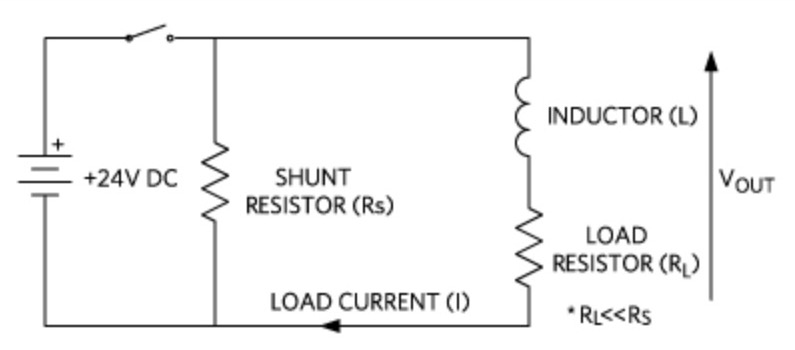##### Figure 1. L-R circuit for DC transient response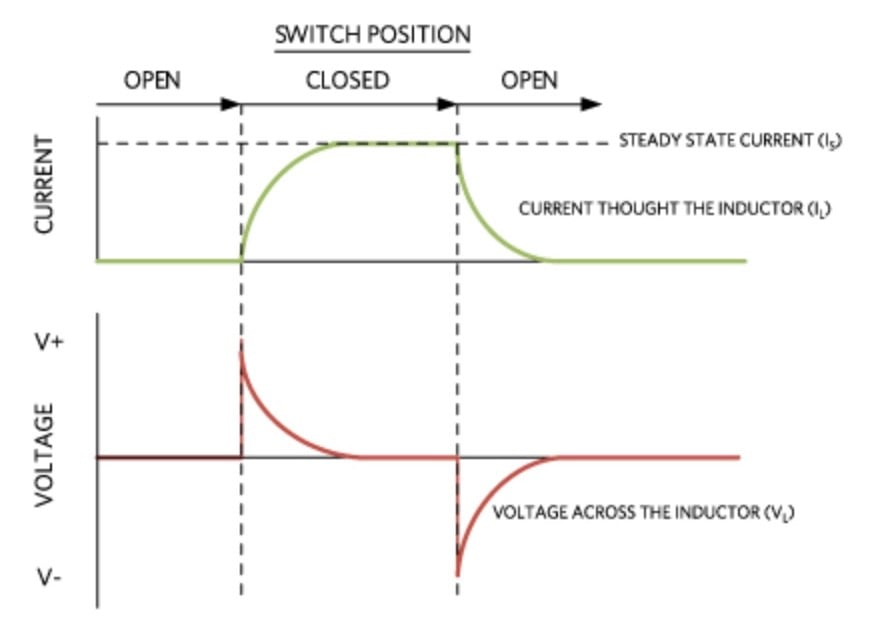##### Figure 2. V-I for an inductive load

1. When the switch is open, no current is flowing and the output or load voltage is 0V.
2. When the switch is closed, current rises exponentially (limited by the back electro-motive force (EMF) of opposite polarity to the supply produced by the inductor) to a steady-state value. The system output voltage is defined by $$V_{OUT} = I \times R_L + \frac{Ldi}{dt}$$ and initially, when the switch closes (and the current is zero), the voltage across the inductor (VL) rises to the supply voltage. As current increases VL decays until a steady-state current (when $$\frac{di}{dt}$$ = 0) is reached where $$Is = \frac{V_{SUPPLY}}{R}$$.
3. When the switch opens, the current decays exponentially toward zero.
4. At the moment the switch opens, the change in current causes a back EMF to be generated. This back EMF has opposite polarity to the current flow, resulting in VL having a negative voltage spike. As current decays to zero, the negative voltage across the inductor returns to 0V.

In a practical circuit, the most common solution to discharge the inductive load (Figure 3), uses a free-wheel diode. In this circuit, while the switch is closed, the diode is reverse-biased and does not conduct any current. When the switch opens, the negative voltage across the inductor forward biases the diode, allowing the stored energy to decay by conducting the current through the diode until steady state is reached and the current is zero.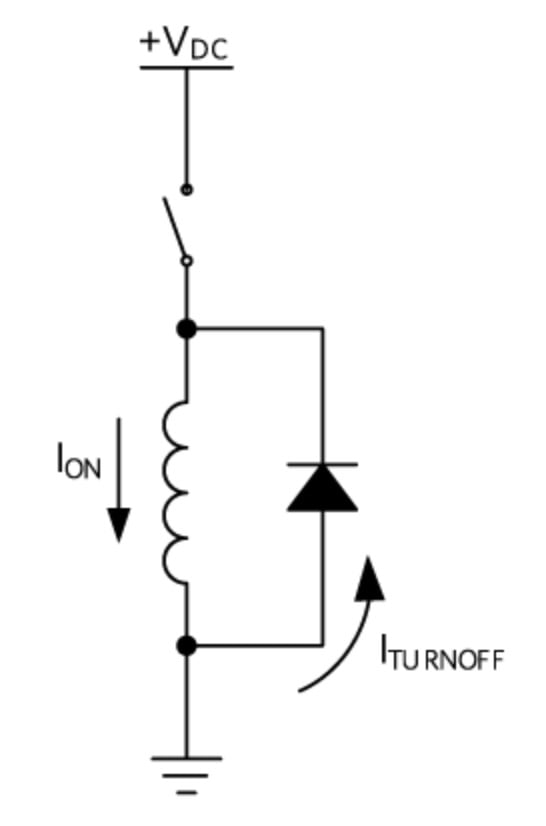##### Figure 3. Freewheel diode

The diode must be able to handle the initial current at turnoff, which equals the steady-state current flowing through the inductor when the switch is closed. In addition, the voltage rating for the diode needs to handle the swing between positive- and negative-voltage levels. A rule of thumb(1) is to select a diode rated for at least the amount of current the inductor coil draws and at least twice the voltage rating of the operating voltage of the load. For many applications, especially those found in industrial applications that have many output channels per IO card, this diode is often physically quite large and adds significant extra cost to the BOM.

The other major disadvantage of the simple freewheel diode approach is that it lengthens the decay of current through the inductor. As explained in "Coil Suppression Can Reduce Relay Life,"(2) this slow decay of current can create problems such as "sticking" between relay contacts. For applications where the current must decay quicker, an alternative solution is to use a Zener diode as shown in Figure 4, which gives a faster current ramp rather than an exponential decay. When the switch opens, the current is shunted through the general-purpose diode and Zener diode path, maintaining a voltage equal to the Zener voltage (plus forward diode drop) until the inductor energy is dissipated.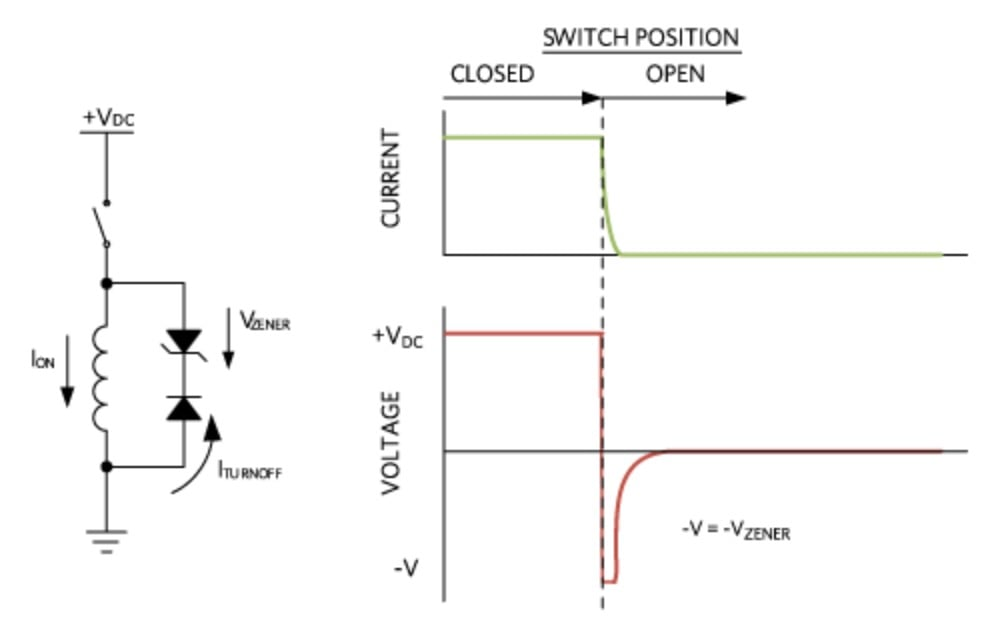### Active Clamping Using MOSFETs

For industrial applications, the 'switch' is usually a MOSFET. When a MOSFET turns off while switching an inductive load, if no protection is available, the voltage across the drain and the source (VDS) increases until the MOSFET breaks down. Modern high-side switches frequently use a technique called active clamping that limits VDS when switching inductive loads to protect the MOSFET. When the switch is closed the MOSFET operates fully on in saturation mode (RDS is low), but when the switch is opened, the MOSFET is driven into its linear mode where RDS is higher resistance. The load demagnetizes quickly during the active clamp because a larger voltage (VDD - VCLAMP) dissipates the stored energy (please refer to "DT99-4: Intelligent Power Switches (IPS): Basic Features & Protection.").(4) The larger the voltage difference, the faster the demagnetization; this is why switch IC vendors often refer to this feature as "fast demag."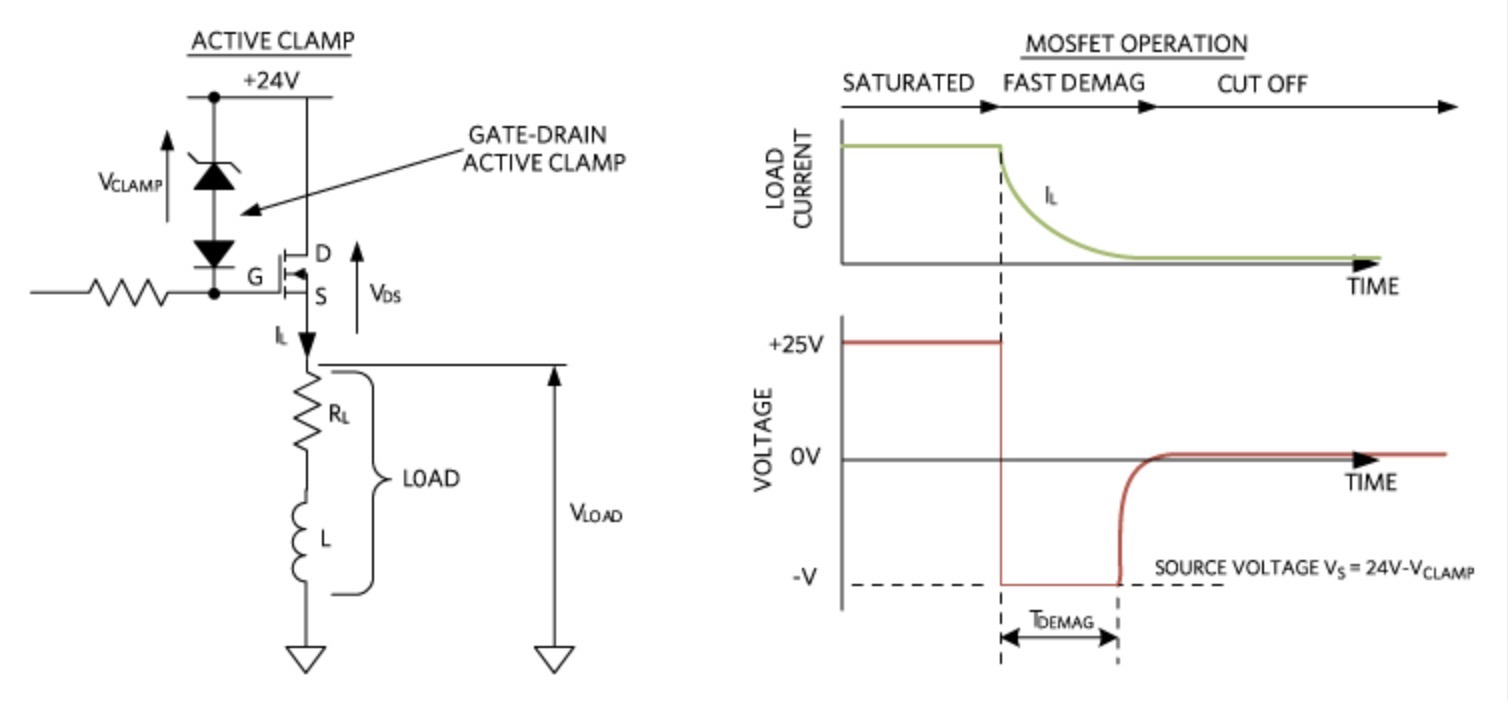##### Figure 5. High-side switch (MOSFET) with active clamp

During demagnetization the MOSFET dissipates more power than the load since the voltage across the MOSFET is higher than the load voltage. This means that for each switch there is a maximum inductive load and load current that can be supported; otherwise, the MOSFET will have thermal issue during active clamp mode. Switch vendors often include a graph in their datasheet to show the maximum inductive load versus inductive current that can be safely handled.

### Demagnetization Energy

Equation 1 defines the energy stored in an inductive load, and Equation 2 defines the energy dissipated by the high-side switch:

$$ELOAD = \frac{1}{2} \times L \times I_{L}^2$$ energy stored in an inductive load

##### Equation 1

$$ESWITCH = \frac{1}{2} \times L \times I_{L}^2 \times (\frac{V_{CLAMP}}{V_{CLAMP} - V_{DD}})$$ energy dissipated by the switch(3)

##### Equation 2

where L is the inductance in Henries and IL is the load current in Amps. During the period the MOSFET is de-energizing the inductive load, the equivalent circuit is shown in Figure 6, where the Zener effectively clamps VDS while a feedback loop controls the gate-source voltage controlling the MOSFET independent of the load current. This way the larger voltage is dropped across the MOSFET (rather than the load) causing the MOSFET to dissipate more energy (and heat) during fast-demag mode. Once the energy has dissipated the load current trends to zero and the MOSFET goes to the cut-off mode and VS trends to 0V.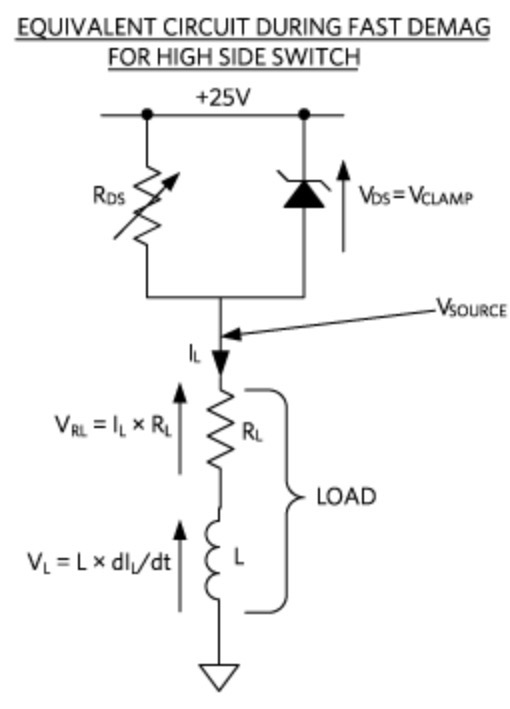##### Figure 6. Equivalent circuit during fast demagnetization for a high-side switch

It is the responsibility of the system designer to ensure that the switch (MOSFET) can handle the higher power that is dissipated during the turn-off mode; otherwise, the increase in junction temperature can cause stress and possible damage to the switch device. This condition is worse for multi-channel switches that are popular in industrial control applications.

### Safe Demagnetization

Although high-side switches typically have overcurrent and overtemperature detection features, during active clamp mode (fast demag) the current is controlled by the energy in the load, so no protection (current or temperature) is active during this mode. To solve the issue of excessive energy dissipation during fast demag and the thermal issues of the MOSFET, Maxim has implemented a new architecture called safe demagnetization (SafeDemag) in the MAX14912 and MAX14913 Octal High-Speed Switch products. SafeDemag works in conjunction with the fast demag circuitry and allows the MAX14912 and MAX14913 to safely turn off loads with unlimited inductance. Under normal turn-off the high-side MOSFET works in linear mode to dissipate the inductor energy using the fast demag feature. If the energy in the inductor, and hence the demagnetization current is too high, the high-side MOSFET begins to overheat. At this point, an on-chip temperature sensor alerts the control logic to turn off the high-side MOSET and turn on the low-side MOSFET providing a low voltage (and hence low power) alternative path for the demagnetization current, allowing the high-side MOSFET to cool and return to safe operating limits.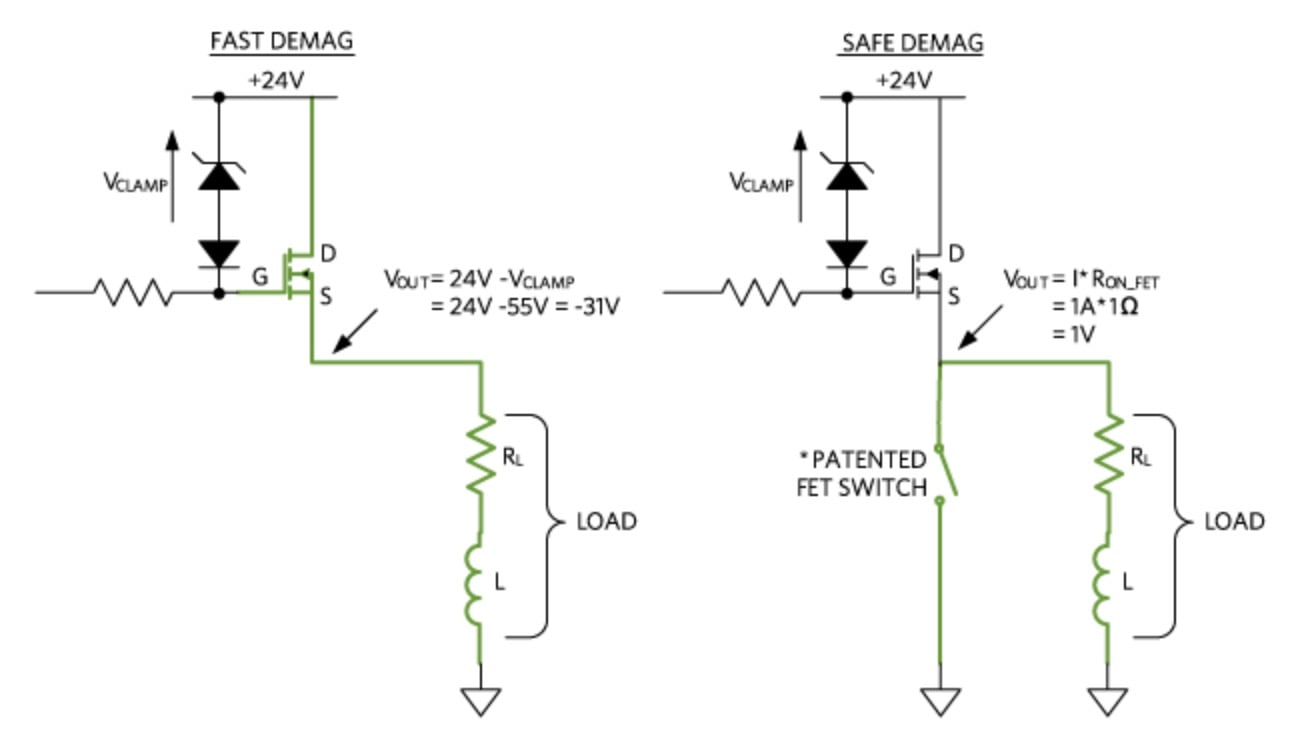##### Figure 7. Current paths for safe demagnetization using low-side MOSFET

UL 508 "Industrial Control Equipment" standard is a standard that defines the requirements for industrial control devices and specifies a maximum load of 48Ω and 1.15H. For the tests in this application note, this standard load demonstrates and compares the performance of various high-side switch products with the different demagnetization schemes already discussed in this application note. All of the switch products are octal-channel devices and one channel is used for the test as shown in Figure 8 to demonstrate the benefit of higher clamp voltage for fast demagnetization vs. 'slow demagnetization' using free-wheel diodes.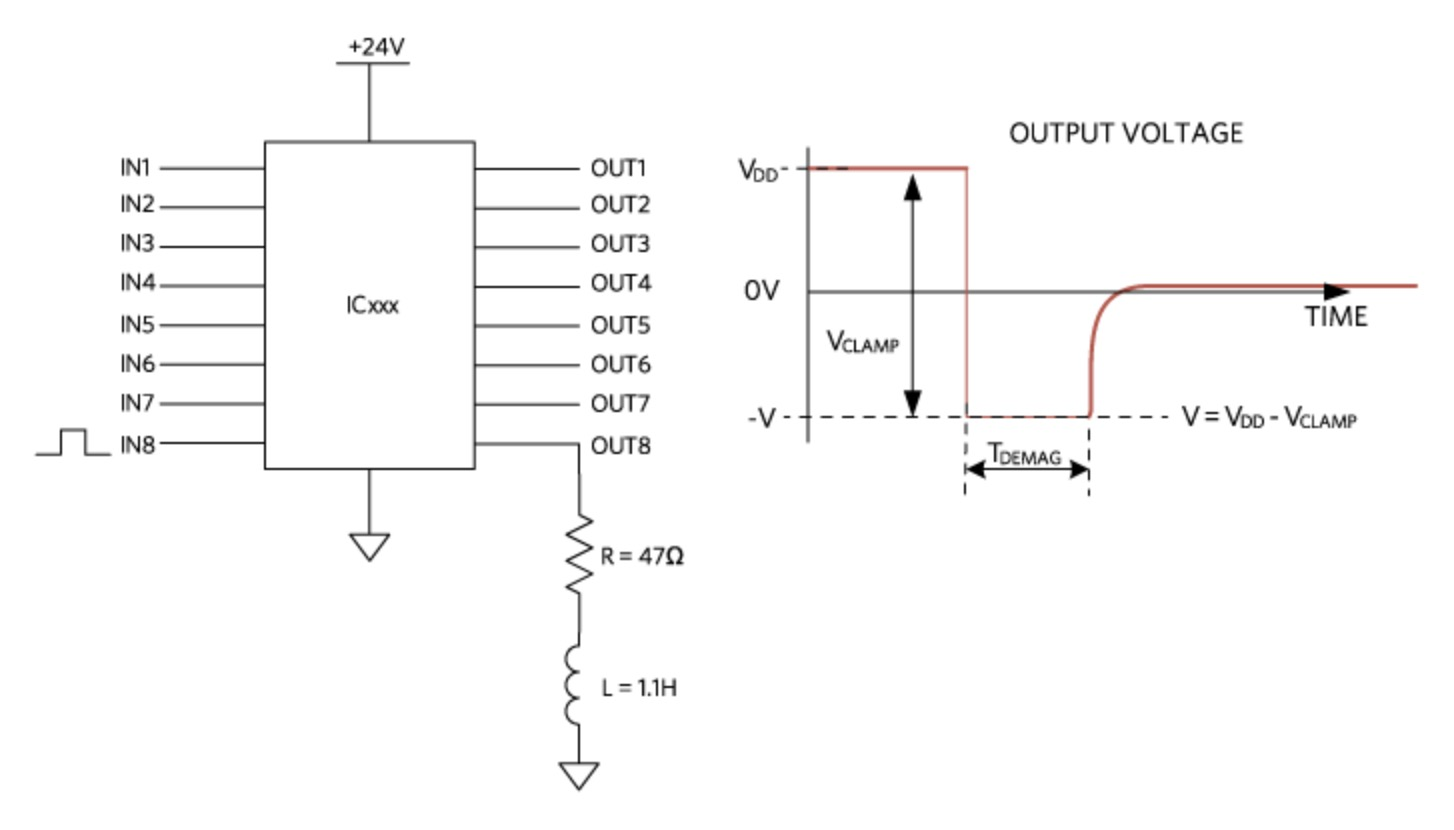##### Figure 8. Test circuit for switching inductor loads for one channel

The formulae for the energy dissipated for a single output channel during demagnetization derives from Equations 3–6:

$$t_{DEMAG} = \frac{L}{R_{LOAD}} \times ln (1 + \frac{V_{DD}}{V_{CLAMP} - V_{DD}})$$

##### Equation 3

$$EOFF = \frac{V_{CLAMP}}{R_{LOAD}} \times (\frac{L \times V_{DD}}{R_{LOAD}} - ((V_{CLAMP} - V_{DD}) \times t_{DEMAG}))$$

##### Equation 4

$$Pt_{OFF} = \frac{EOFF}{t_{DEMAG}}$$

##### Equation 5

$$Pt_{OUT} = \frac{V_{DD}}{R_{LOAD}}$$

##### Equation 6

For this analysis, we assume the switch close-opening time is much larger than tDEMAG to allow the energy in the inductor to dissipate and the switch to reach steady state off condition before it is turned on again. Test are conducted using commercially available high-side switch ICs from Maxim and other IC vendors as listed in Table 1.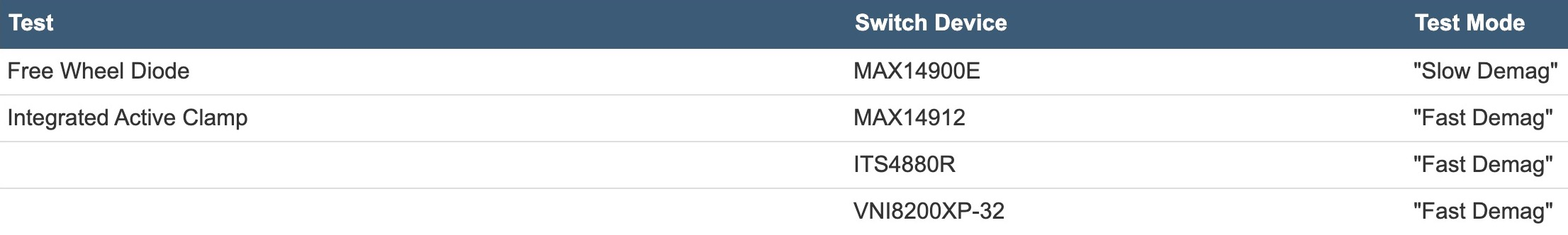#### Test 1: Free Wheel Diode ("Slow Demag")

The MAX14900E evaluation kit operating in parallel mode, uses two MURA205T3G diodes connected from each OUTPUT channel to VDD and GND to implement a free-wheel diode scheme. The input is a 1Hz square wave. Figure 9 shows the waveforms—Channel 1 (yellow) is the input signal, Channel 2 (magenta) is the output voltage, and Channel 4 (green) is the inductive load current. As expected the diodes limit the voltage swing to < 1V below ground and the demagnetization is relatively slow at approximately 94ms.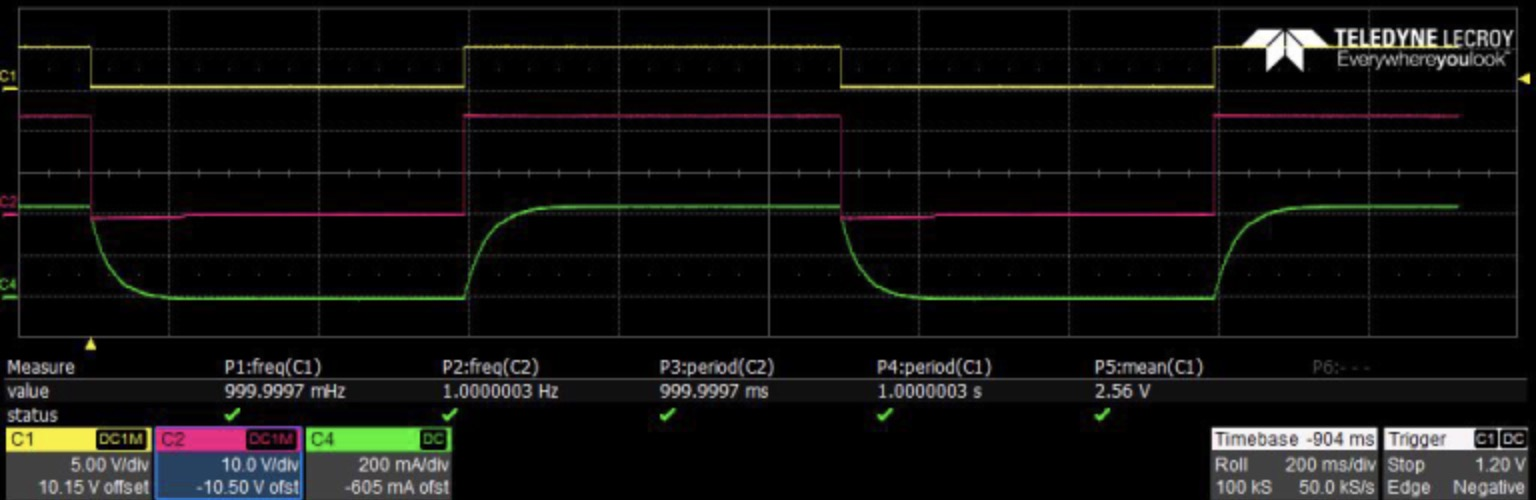#### Test 2: Fast Demagnetization

Three products were used, Maxim's MAX14912 and two competitor products, Infineon's ITS4880R and STM's VNI8200XP. All switches are operated in parallel mode with a 1Hz square-wave input. Figures 10, 11, and 12 show the waveforms for the MAX14912, ITS4880, and VNI8200, respectively. In each case Channel 1 (yellow) is the input signal, Channel 2 (magenta) is the output voltage, and Channel 4 (green) is the inductive load current. The first scope shot shows the clamp voltage and the second scope shot the demag time.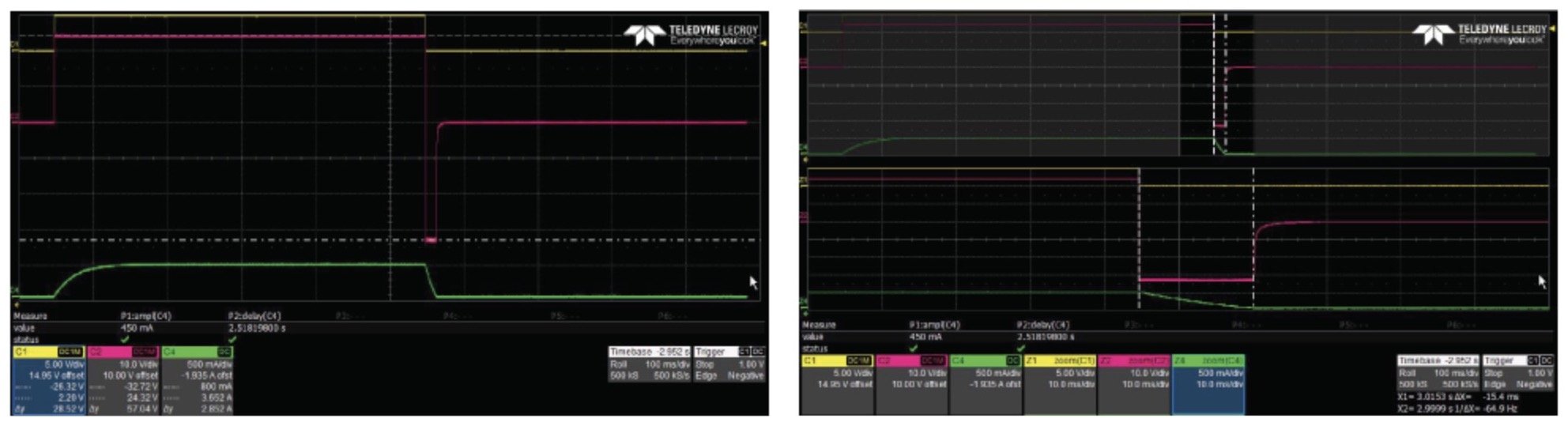##### Figure 10. MAX14912 with fast demag (A - VCLAMP, B - tDEMAG)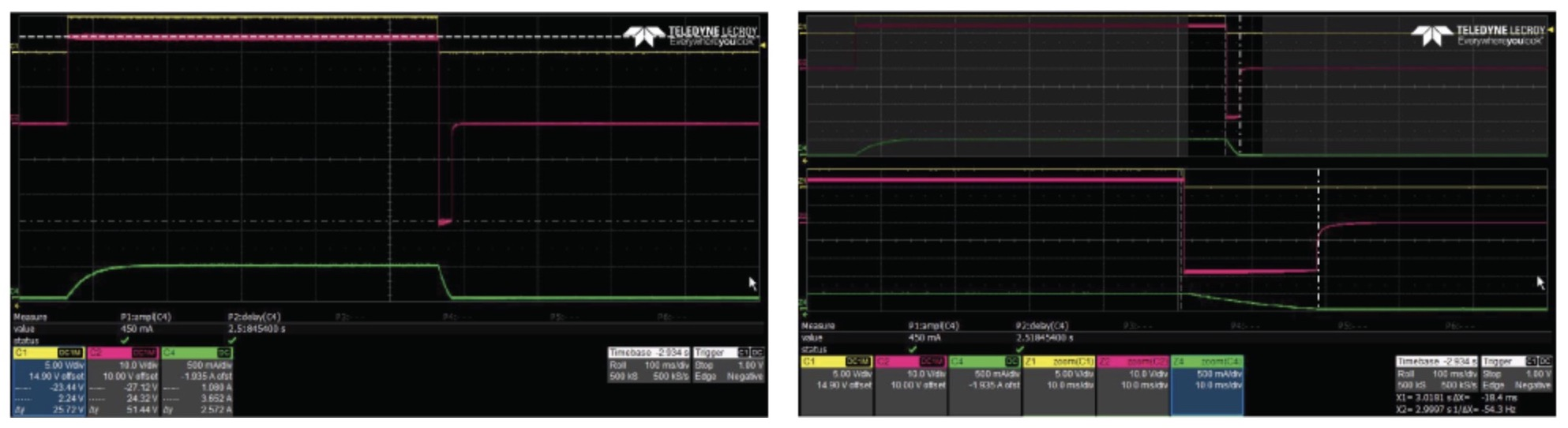##### Figure 11. ITS4880 with fast demag (A - VCLAMP, B - tDEMAG)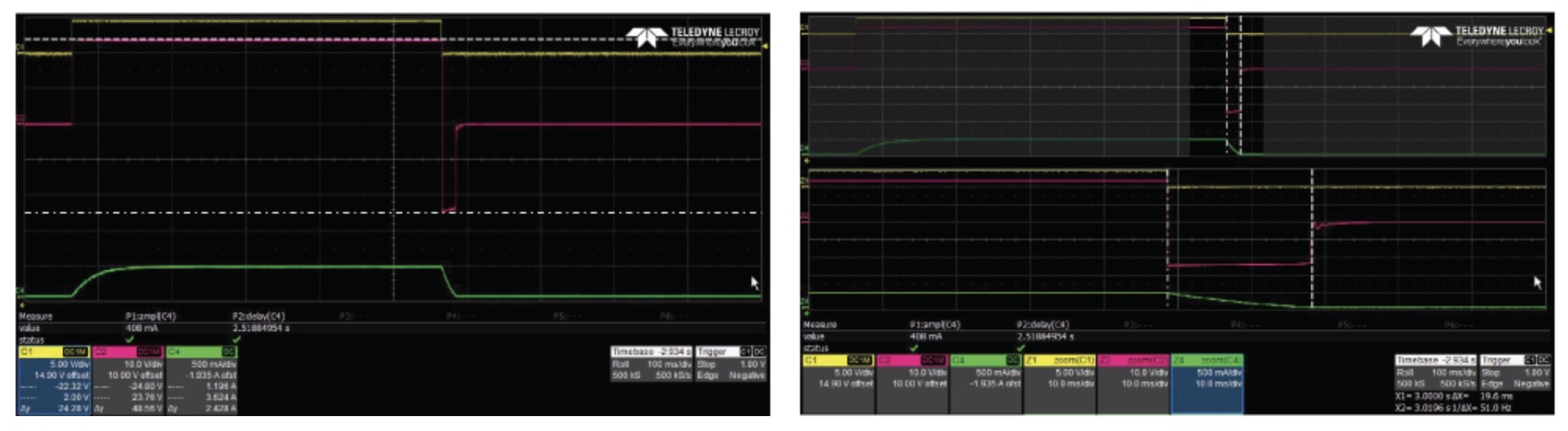#### Summary for Fast Demagnetization

As expected, the fast demag function provides a faster demagnetization time than the simple freewheel diode scheme, and the measured values correlate with the calculations from equations 3–6. The higher clamp voltage of the MAX14912 give approximately 20% faster demagnetization than the competitor products.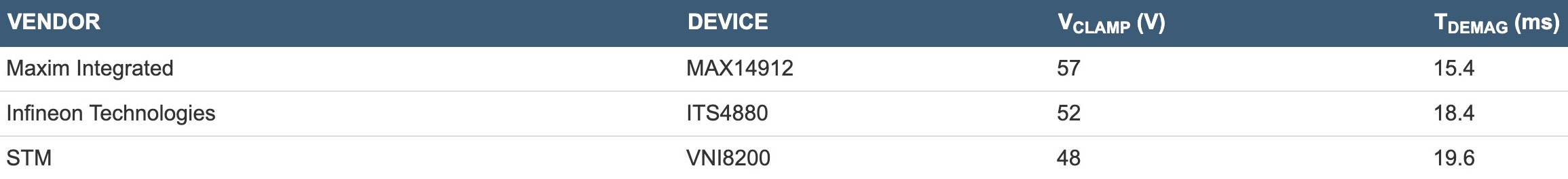#### Test 3: Safe Demagnetization (MAX14912)

In order to stress the switch, all eight-output channels were switched simultaneously. The load for each output is 1.5H and 27Ω. The inputs are driven from a common input signal, which is a 2Hz square wave. The test circuit is shown in Figure 13.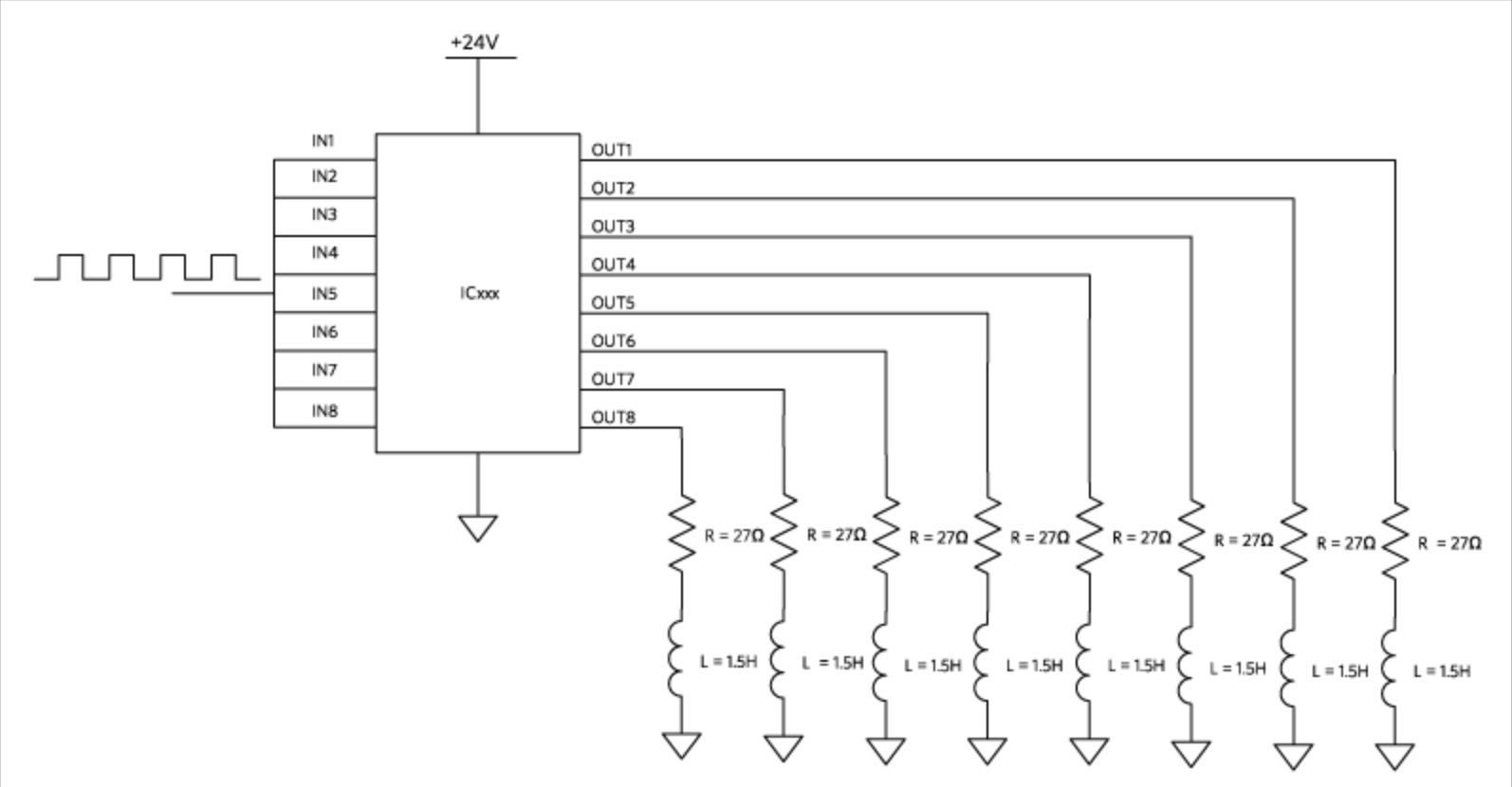##### Figure 13. Test circuit for switching inductor loads for all eight channels simultaneously

All tests are at room temperature with a 24V power supply. The scope shots for ITS4880R in Figure 14a and 14b shows the waveforms—Channel 1 (yellow) is the input signal, Channel 2 (magenta) is the output voltage, Channel 3 (teal) is the overtemperature warning pin, and Channel 4 (green) is the inductive load current.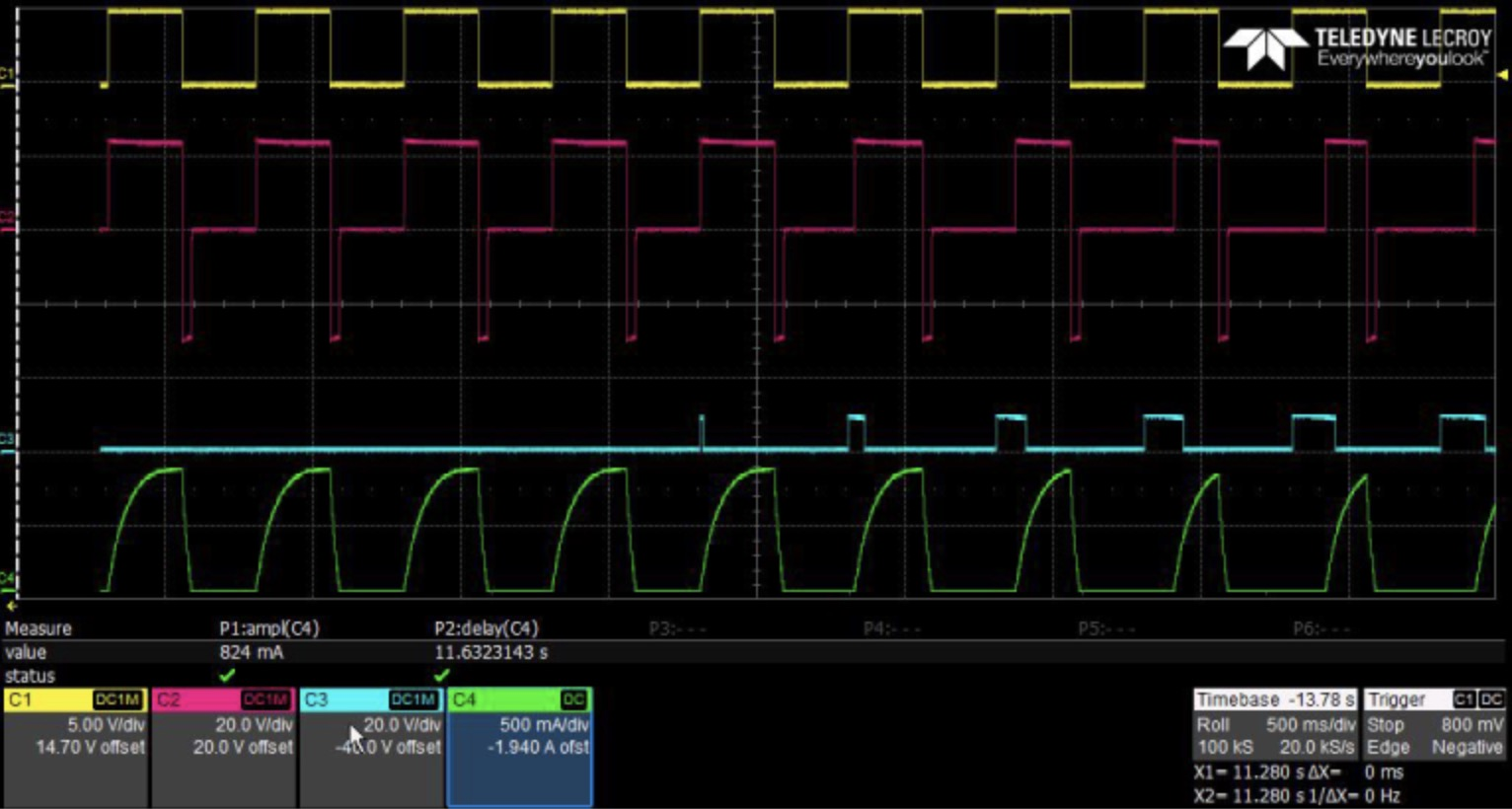##### Figure 14a. ITS4880R switching inductor loads for all eight channels simultaneously

After a few seconds operation, the ITS4880R starts to overheat and the overtemperature warning pin is driven high in the period when the input is high and the output is low. The protection turns on when the device is over temperature, then after a number of milliseconds of cooling, it turns back on.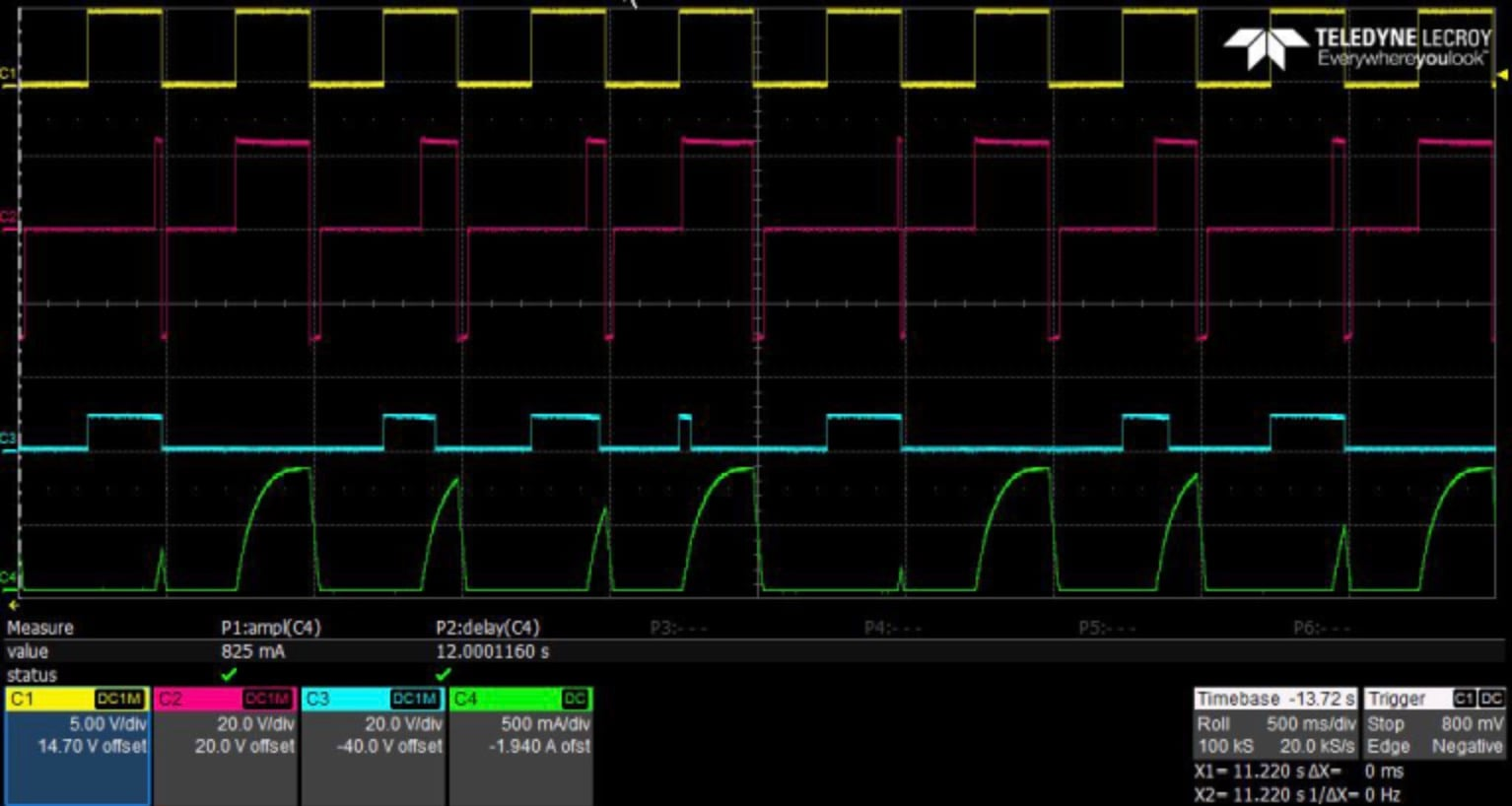##### Figure 14b. ITS4880R switching inductor loads for all eight channels simultaneously

After a few more seconds, the impact of overtemperature protection can be seen more clearly from the inductor current waveform shown in Figure 14b. There are two issues arising from the output switch being turned off to protect from overheating; the first is less time for demag (potentially failing to fully discharge the inductor), and the second is insufficient time for the inductor to fully charge (potentially failing to operate correctly in the case of a device such a solenoid or relay).

The scope shots for MAX14912 in Figure 15a, 15b, and 15c show the waveforms—Channel 1 (yellow) is the input signal, Channel 2 (magenta) is the output voltage, and Channel 4 (green) is the inductive load current.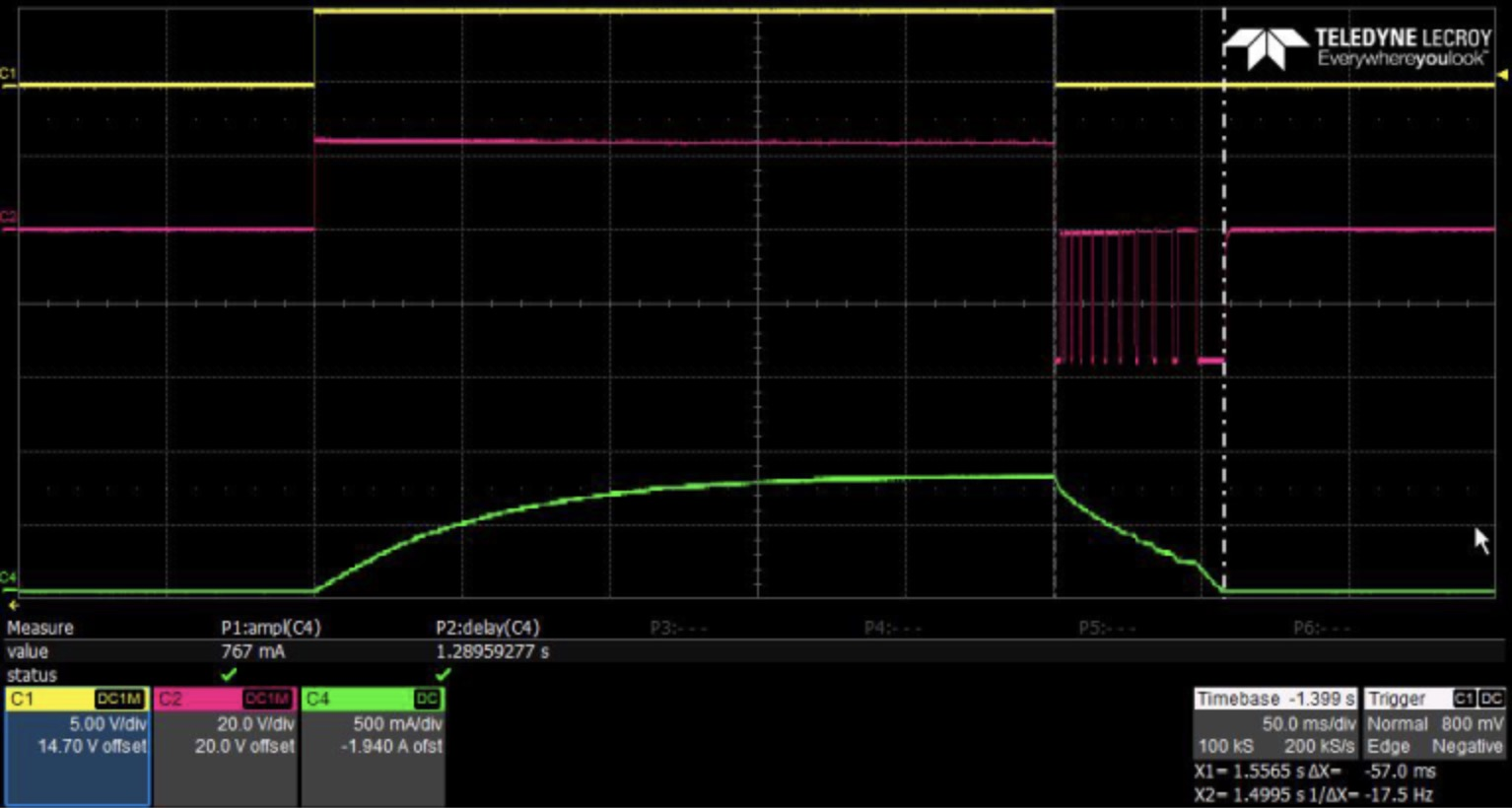##### Figure 15a. MAX14912 switching inductor loads for all eight channels simultaneously

In Figures 15a and 15b the SafeDemag feature can be seen operating. When the fast demag clamp starts to overheat the SafeDemag starts operating, causing the output voltage to return to 0V while the low side switch is bleeding off the inductor energy. As the MAX14912 cools, it returns to high side "fast demag" and the output voltage returns to a negative value as defined by the clamp voltage. The total demag time is increased but the inductor current decays smoothly as the energy is dissipated. The MAX14912 continues to run as shown in Figure 15c with no overheating issues caused by excess inductive loading.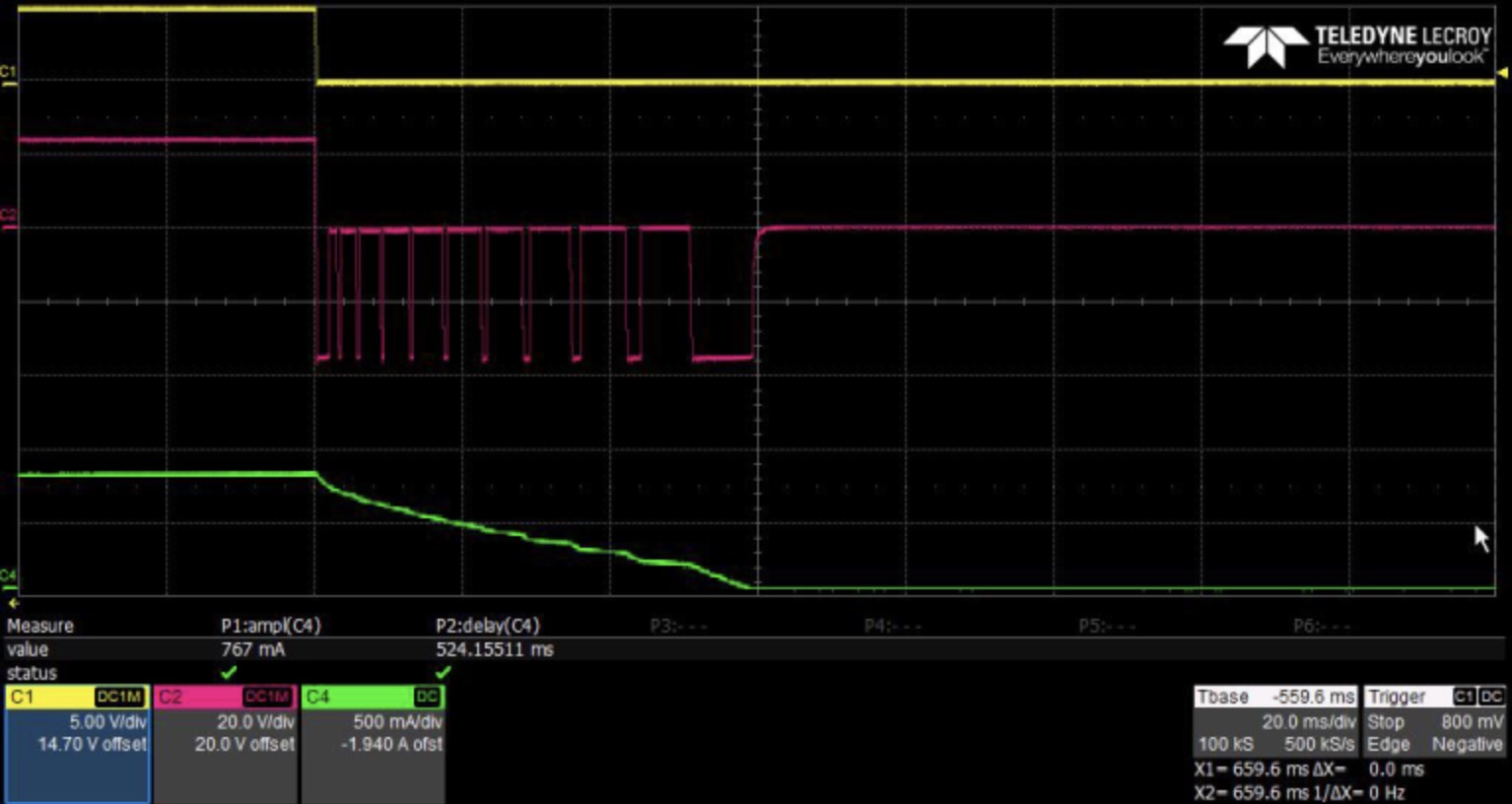##### Figure 15b. MAX14912 switching inductor loads for all eight channels simultaneously with SafeDemag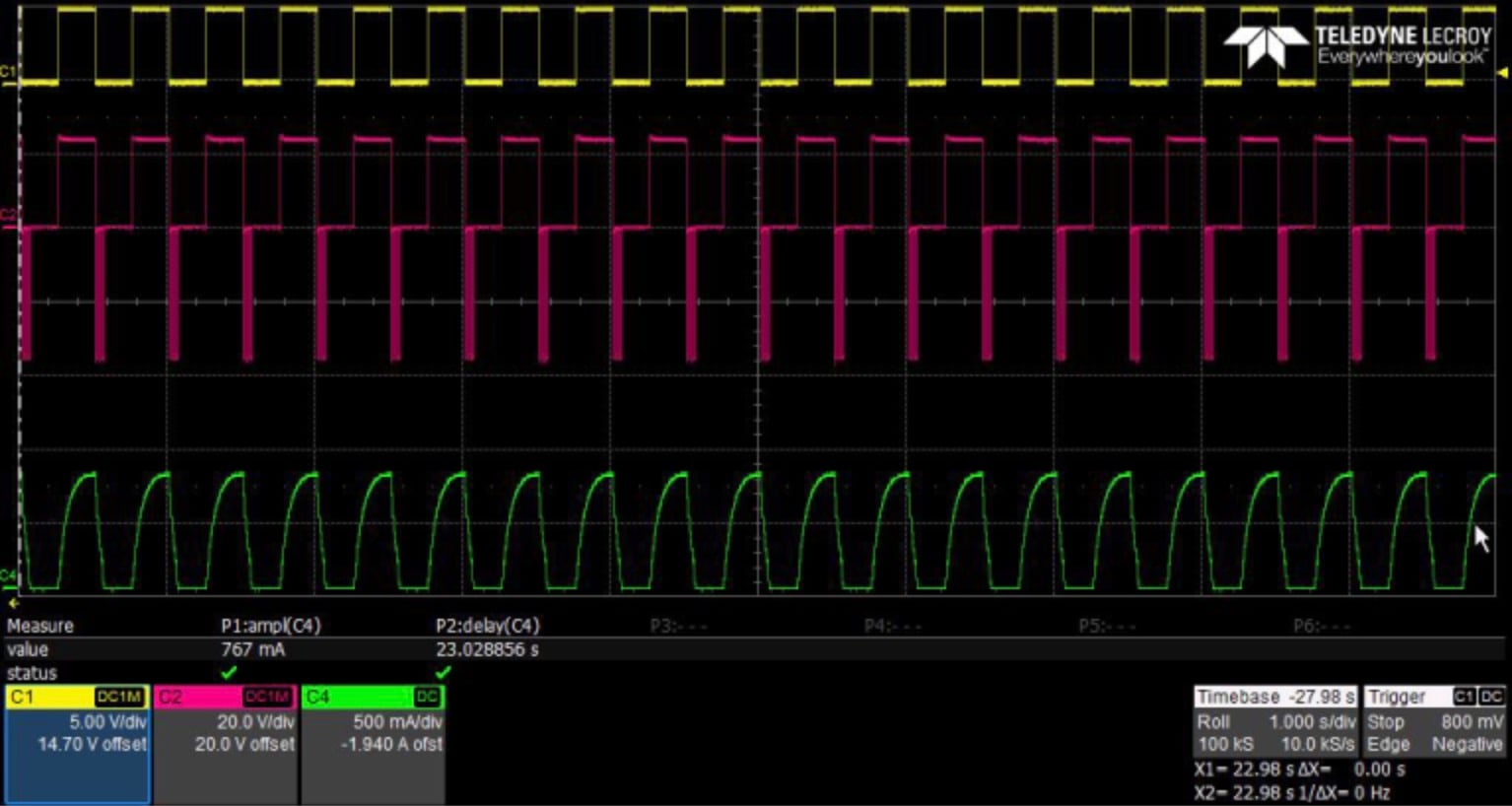##### Figure 15c. MAX14912 switching inductor loads for all eight channels simultaneously running continuously

These tests were conducted at room temperature so the performance of devices without SafeDemag would be even worse when operated at higher temperatures as found in many industrial applications.

### MAX14912/MAX14913 Competitive Comparison Summary

This application note has demonstrated the differentiated features of MAX14912/MAX14913 that provide design engineers with key benefits compared to Maxim's MAX14900 as well as competitor devices such as ITS4880R and VNI8200. MAX14912 has a higher clamp voltage giving a faster demagnetization for inductive loads, and unlike other switches that have a limit for the size of inductance that can be switched, SafeDemag allows MAX14912/MAX14913 to safely switch loads of unlimited inductance. SafeDemag also allows MAX14912/MAX14913 to use a smaller package with up to a 66% reduction is footprint compared to competition.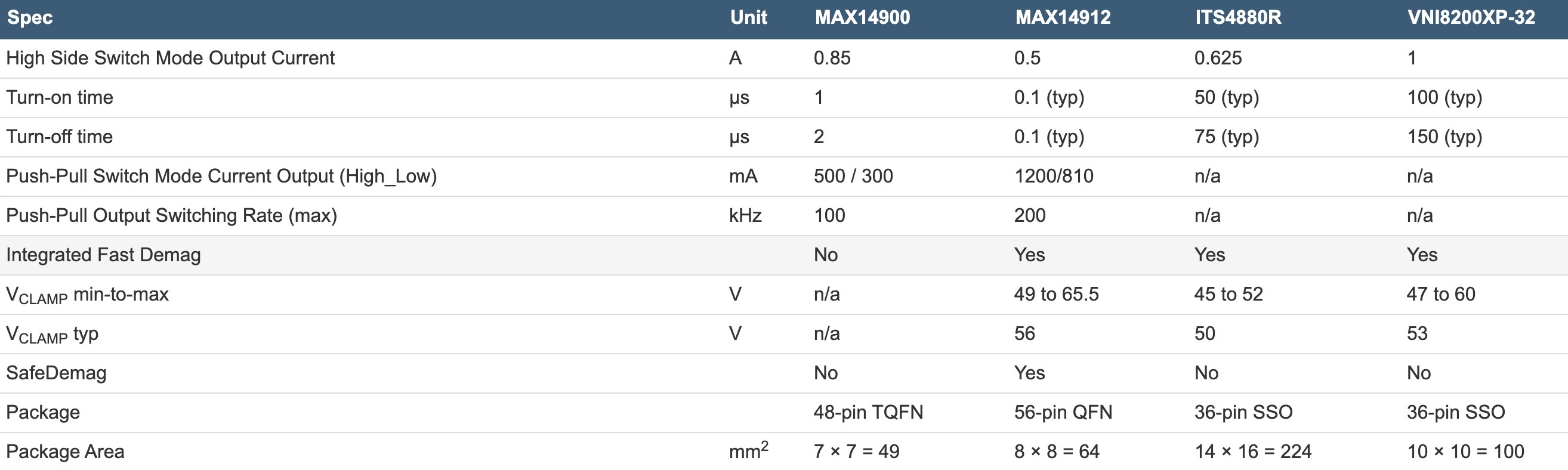### Conclusion

The ability to safely switch inductive loads is critical for industrial applications and system engineers have different solutions to this problem. The data presented in this application note demonstrates that using a freewheel diode for slow demag is good, using an active clamp for fast demag is better, but using Maxim's SafeDemag is the best solution to this problem.

#### References

(1) "Switching Inductive Loads." Pdf. Artisan Controls. N.p., n.d. Web. 08 Dec. 2016.

(2) "Coil Suppression Can Reduce Relay Life." Pdf. TE Connectivity. N.p., n.d. Web. 08 Dec. 2016.

(3) "Application Note (AN4402): Multichannel drivers driving inductive loads with L99MC6 and L9733." Pdf. L9733 - Octal Configurable Low/high Side Driver - STMicroelectronics. N.p., n.d. Web. 08 Dec. 2016.

(4) "Application Note (AN2208): Designing Industrial Applications with VN808/VN340SP High-side Drivers." Pdf. VN808-E - Octal Channel High SideDriver - STMicroelectronics. N.p., n.d. Web. 08 Dec. 2016.

• Share